• Slides: 20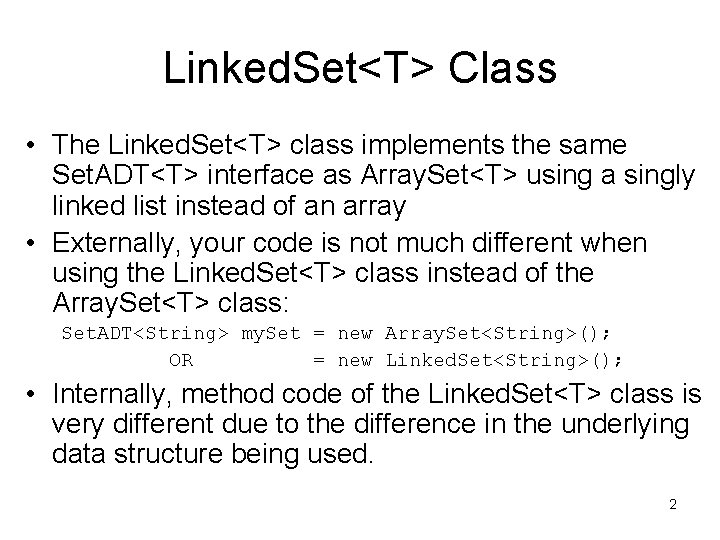Linked. Set<T> Class • Again: We assume that Set. ADT<T> interface extends Iterable. 3Linked. Set Attributes/Constructor • The class definition starts with: public class Linked. Set<T> implements Set. ADT<T> { private static Random rand = new Random(); private int count; private Linear. Node<T> contents; public Linked. Set() { count = 0; contents = null; } // default constructor // NOTE: not an array 4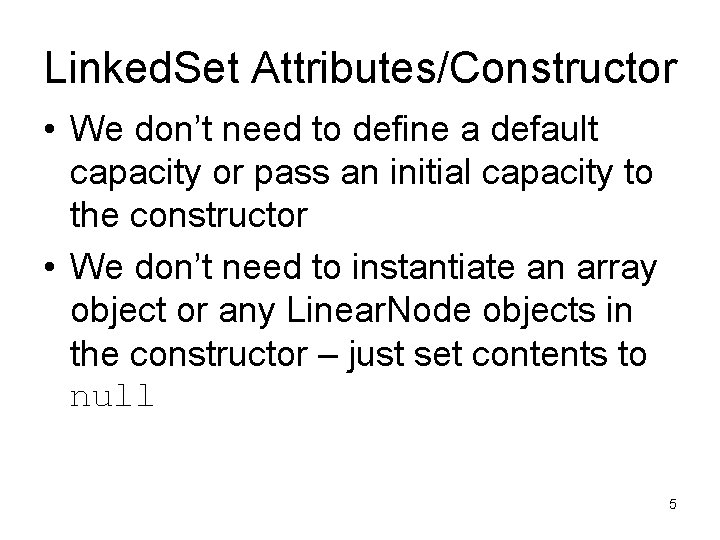Linked. Set Attributes/Constructor • We don’t need to define a default capacity or pass an initial capacity to the constructor • We don’t need to instantiate an array object or any Linear. Node objects in the constructor – just set contents to null 5Linked. Set Methods • Note that because we are not using a fixed size data structure such as an array, we don’t need a private expand. Capacity() method. 6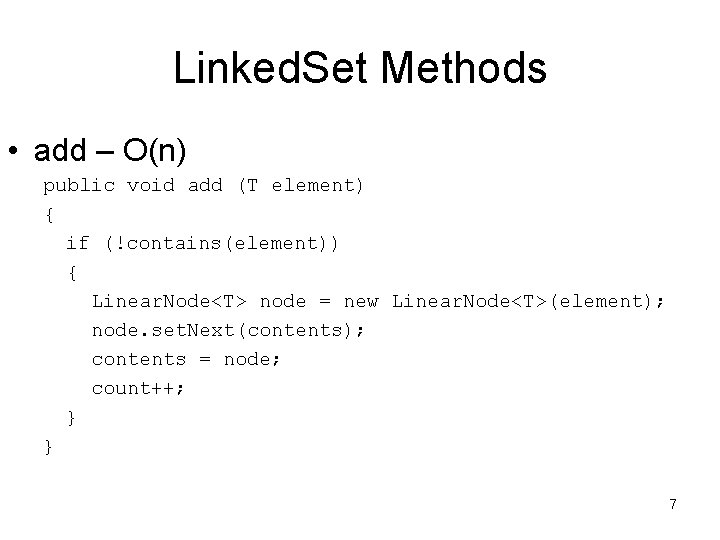Linked. Set Methods • add – O(n) public void add (T element) { if (!contains(element)) { Linear. Node<T> node = new Linear. Node<T>(element); node. set. Next(contents); contents = node; count++; } } 7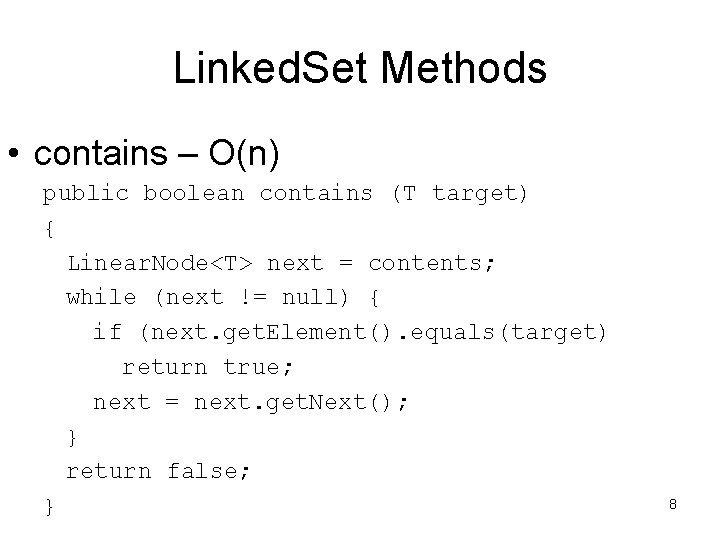Linked. Set Methods • contains – O(n) public boolean contains (T target) { Linear. Node<T> next = contents; while (next != null) { if (next. get. Element(). equals(target) return true; next = next. get. Next(); } return false; } 8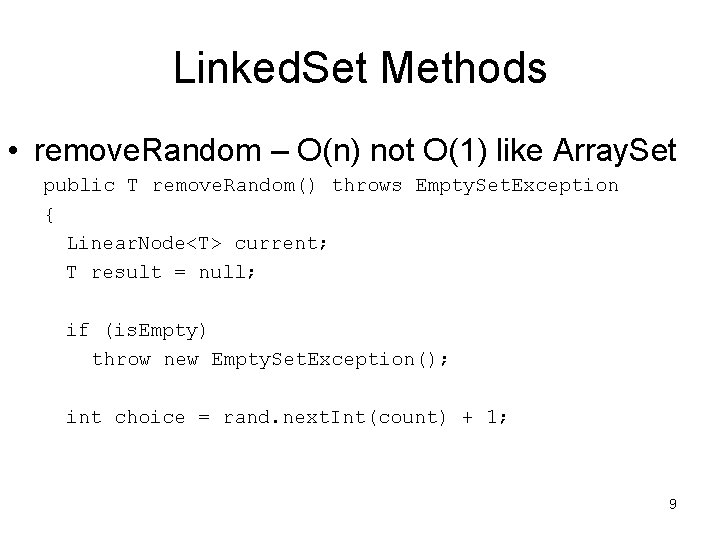Linked. Set Methods • remove. Random – O(n) not O(1) like Array. Set public T remove. Random() throws Empty. Set. Exception { Linear. Node<T> current; T result = null; if (is. Empty) throw new Empty. Set. Exception(); int choice = rand. next. Int(count) + 1; 9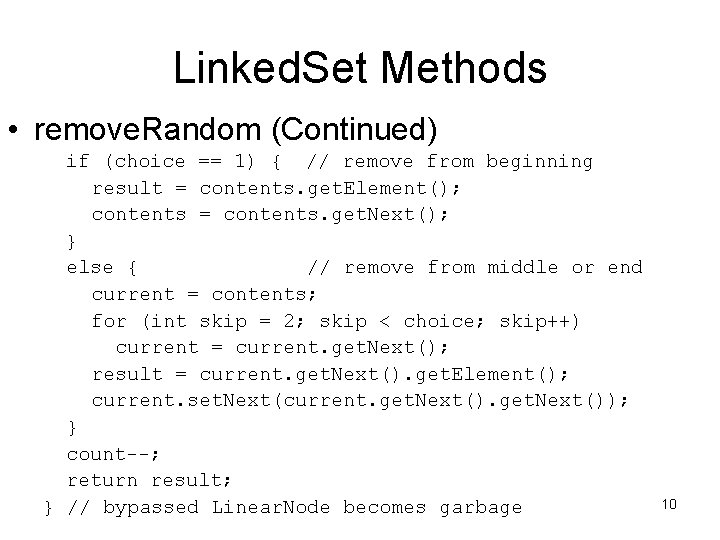Linked. Set Methods • remove. Random (Continued) if (choice == 1) { // remove from beginning result = contents. get. Element(); contents = contents. get. Next(); } else { // remove from middle or end current = contents; for (int skip = 2; skip < choice; skip++) current = current. get. Next(); result = current. get. Next(). get. Element(); current. set. Next(current. get. Next()); } count--; return result; } // bypassed Linear. Node becomes garbage 10Linked. Set iterator Method • iterator – O(1) public Iterator<T> iterator { return new Linked. Iterator<T>(contents) } • We will study the Linked. Iterator class to understand how it is implemented 11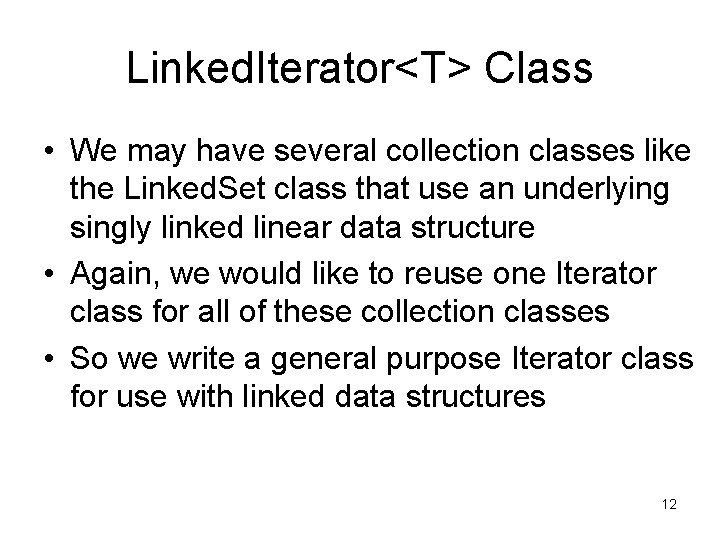Linked. Iterator<T> Class • We may have several collection classes like the Linked. Set class that use an underlying singly linked linear data structure • Again, we would like to reuse one Iterator class for all of these collection classes • So we write a general purpose Iterator class for use with linked data structures 12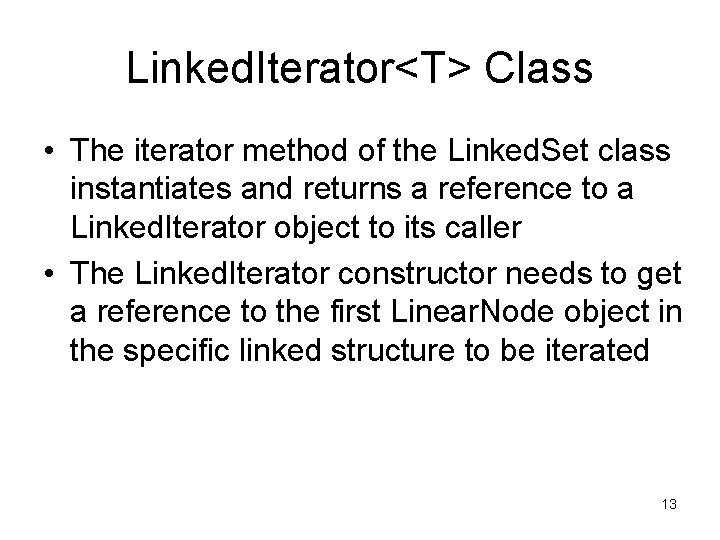Linked. Iterator<T> Class • The iterator method of the Linked. Set class instantiates and returns a reference to a Linked. Iterator object to its caller • The Linked. Iterator constructor needs to get a reference to the first Linear. Node object in the specific linked structure to be iterated 13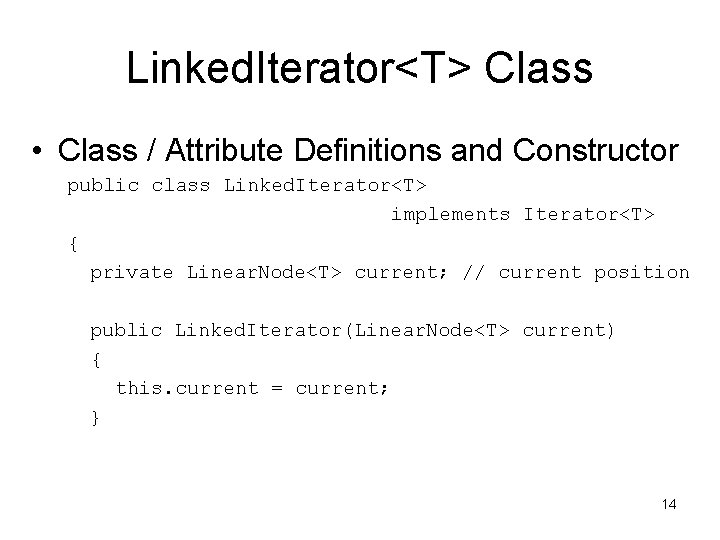Linked. Iterator<T> Class • Class / Attribute Definitions and Constructor public class Linked. Iterator<T> implements Iterator<T> { private Linear. Node<T> current; // current position public Linked. Iterator(Linear. Node<T> current) { this. current = current; } 14Linked. Iterator Methods • has. Next – O(1) public boolean has. Next() { return current != null; } • next – O(1) public T next() { if (!has. Next()) throw new No. Such. Element. Exception(); T result = current. get. Element(); current = current. get. Next(); return result; 15 } // old Linear. Node does not become garbage (Why? )Linked. Iterator Methods • remove – O(1) • We don’t need to implement real code for the remove method, but there is no return value that we can use to indicate that it is not implemented • If we don’t implement it, we indicate that the code is not implemented by throwing an exception public void remove() throws Unsupported. Operation. Exception { throw new Unsupported. Operation. Exception(); } 16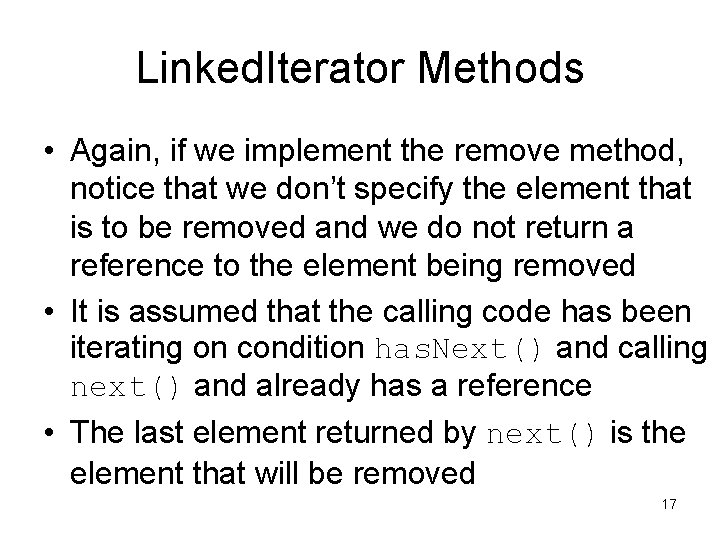Linked. Iterator Methods • Again, if we implement the remove method, notice that we don’t specify the element that is to be removed and we do not return a reference to the element being removed • It is assumed that the calling code has been iterating on condition has. Next() and calling next() and already has a reference • The last element returned by next() is the element that will be removed 17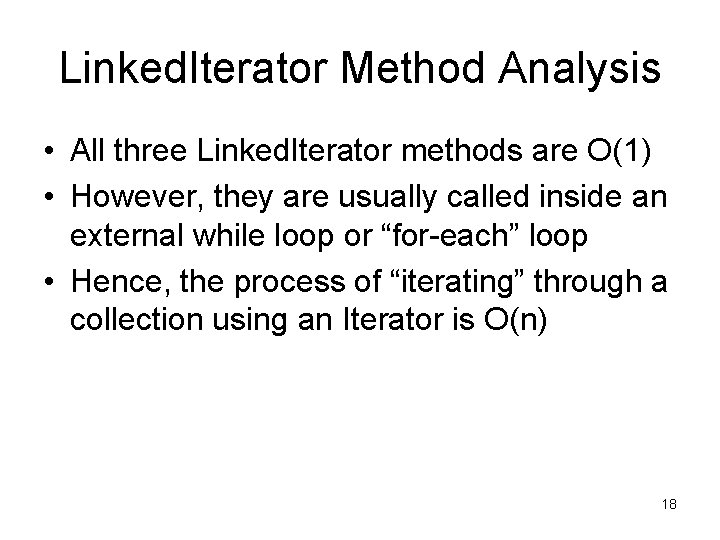Linked. Iterator Method Analysis • All three Linked. Iterator methods are O(1) • However, they are usually called inside an external while loop or “for-each” loop • Hence, the process of “iterating” through a collection using an Iterator is O(n) 18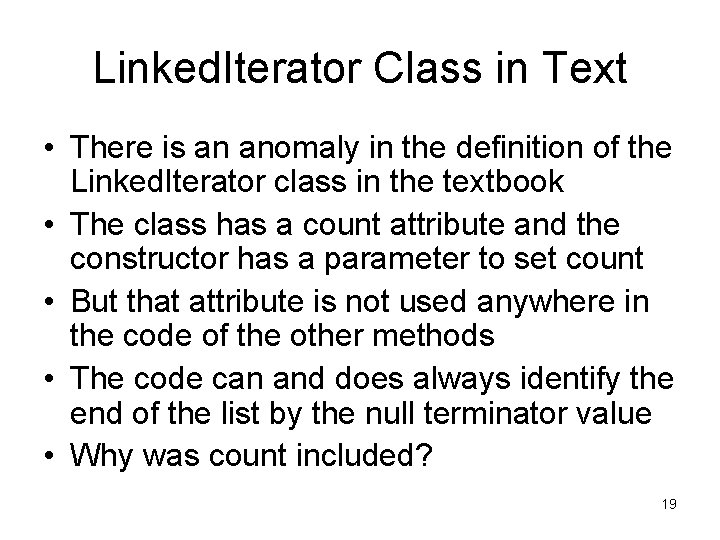Linked. Iterator Class in Text • There is an anomaly in the definition of the Linked. Iterator class in the textbook • The class has a count attribute and the constructor has a parameter to set count • But that attribute is not used anywhere in the code of the other methods • The code can and does always identify the end of the list by the null terminator value • Why was count included? 19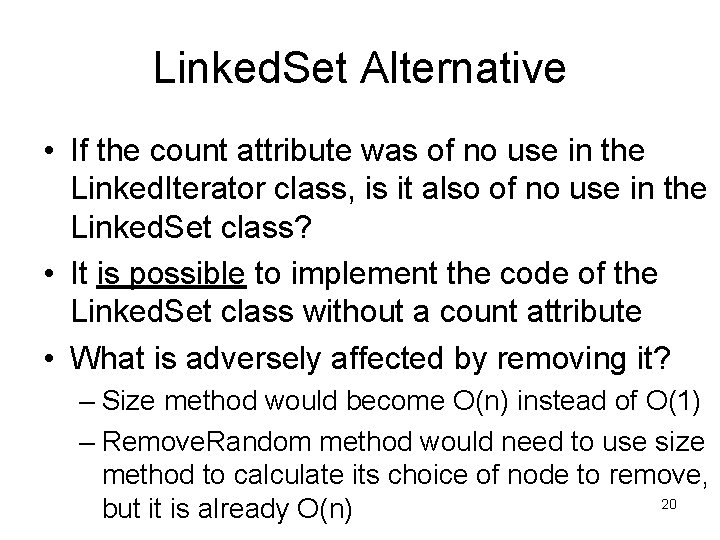Linked. Set Alternative • If the count attribute was of no use in the Linked. Iterator class, is it also of no use in the Linked. Set class? • It is possible to implement the code of the Linked. Set class without a count attribute • What is adversely affected by removing it? – Size method would become O(n) instead of O(1) – Remove. Random method would need to use size method to calculate its choice of node to remove, 20 but it is already O(n)